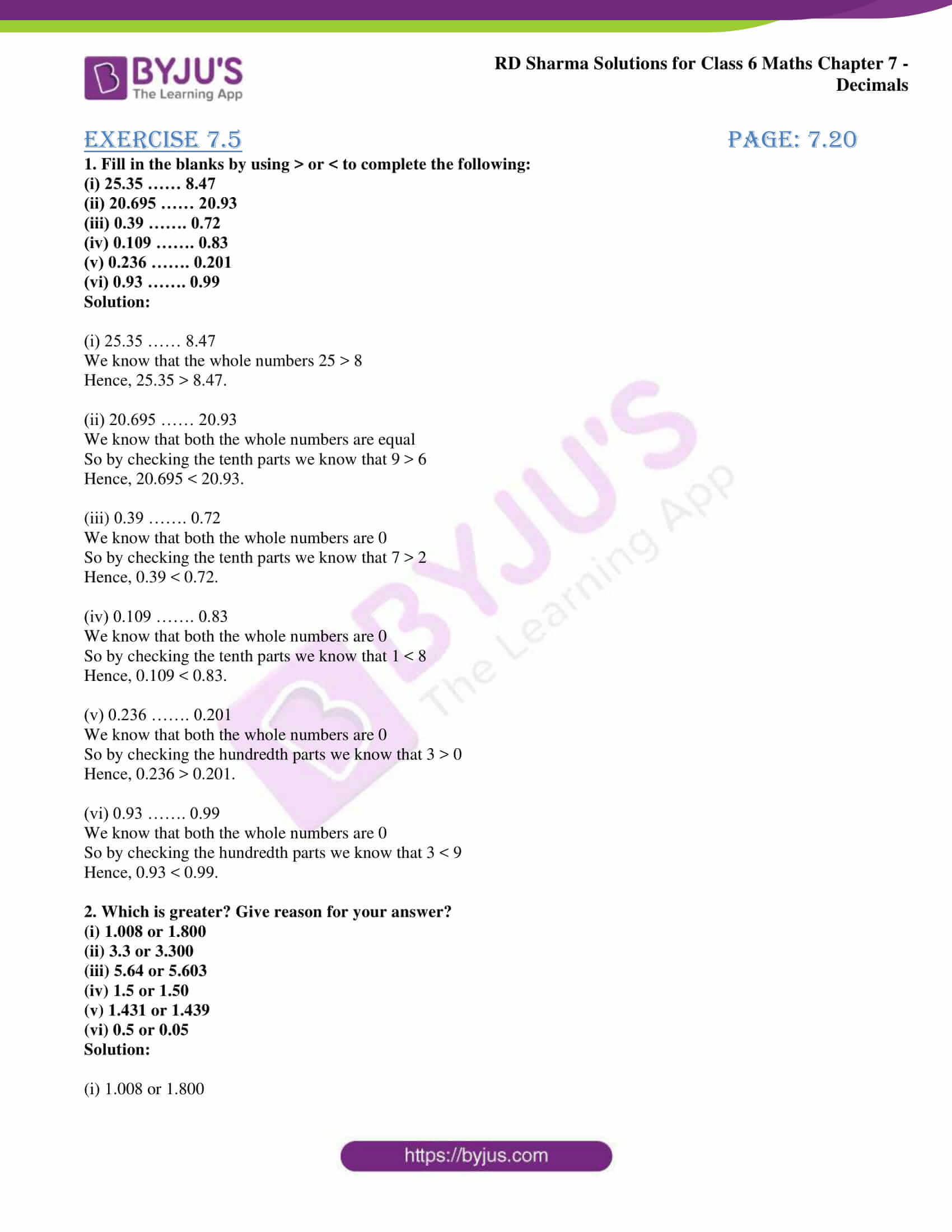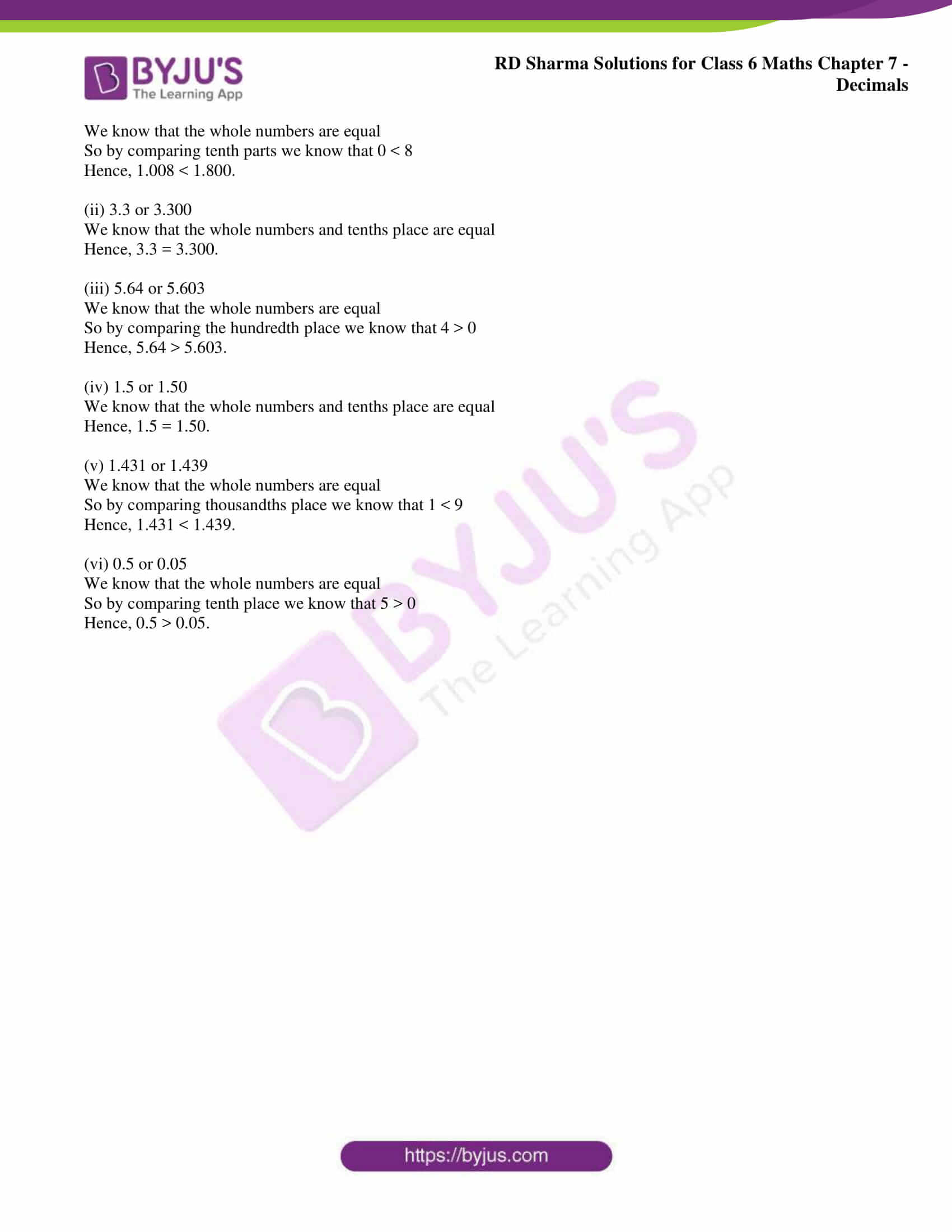# RD Sharma Solutions for Class 6 Chapter 7: Decimals Exercise 7.5

The solutions prepared by faculty at BYJU’S help students to get an overall idea about the concepts which are covered in each exercise. This exercise helps students understand the steps followed in comparing the given set of decimals. RD Sharma solutions Class 6 improves logical thinking capacity among students. The exercise wise problems of the textbook can be solved with the help of RD Sharma Solutions for Class 6 Maths Chapter 7 Decimals Exercise 7.5 PDF which are provided here.

## RD Sharma Solutions for Class 6 Chapter 7: Decimals Exercise 7.5 Download PDF### Access RD Sharma Solutions for Class 6 Chapter 7: Decimals Exercise 7.5

1. Fill in the blanks by using > or < to complete the following:

(i) 25.35 …… 8.47

(ii) 20.695 …… 20.93

(iii) 0.39 ……. 0.72

(iv) 0.109 ……. 0.83

(v) 0.236 ……. 0.201

(vi) 0.93 ……. 0.99

Solution:

(i) 25.35 …… 8.47

We know that the whole numbers 25 > 8

Hence, 25.35 > 8.47.

(ii) 20.695 …… 20.93

We know that both the whole numbers are equal

So by checking the tenth parts we know that 9 > 6

Hence, 20.695 < 20.93.

(iii) 0.39 ……. 0.72

We know that both the whole numbers are 0

So by checking the tenth parts we know that 7 > 2

Hence, 0.39 < 0.72.

(iv) 0.109 ……. 0.83

We know that both the whole numbers are 0

So by checking the tenth parts we know that 1 < 8

Hence, 0.109 < 0.83.

(v) 0.236 ……. 0.201

We know that both the whole numbers are 0

So by checking the hundredth parts we know that 3 > 0

Hence, 0.236 > 0.201.

(vi) 0.93 ……. 0.99

We know that both the whole numbers are 0

So by checking the hundredth parts we know that 3 < 9

Hence, 0.93 < 0.99.

(i) 1.008 or 1.800

(ii) 3.3 or 3.300

(iii) 5.64 or 5.603

(iv) 1.5 or 1.50

(v) 1.431 or 1.439

(vi) 0.5 or 0.05

Solution:

(i) 1.008 or 1.800

We know that the whole numbers are equal

So by comparing tenth parts we know that 0 < 8

Hence, 1.008 < 1.800.

(ii) 3.3 or 3.300

We know that the whole numbers and tenths place are equal

Hence, 3.3 = 3.300.

(iii) 5.64 or 5.603

We know that the whole numbers are equal

So by comparing the hundredth place we know that 4 > 0

Hence, 5.64 > 5.603.

(iv) 1.5 or 1.50

We know that the whole numbers and tenths place are equal

Hence, 1.5 = 1.50.

(v) 1.431 or 1.439

We know that the whole numbers are equal

So by comparing thousandths place we know that 1 < 9

Hence, 1.431 < 1.439.

(vi) 0.5 or 0.05

We know that the whole numbers are equal

So by comparing tenth place we know that 5 > 0

Hence, 0.5 > 0.05.